T-SQL

# Modifying data using table valued functions

Is it possible to modify data in T-SQL using inline table valued functions? It´s surprising, but YES, it´s possible. If we will remember the sentence from BOL, that we can think about inline table valued functions as  they are like parameterized views, then it’s more clear that we can modify data using them like we can do it using views. Let us show how it works.

First we will create dbo.SampleTable with simple data inside:

```CREATE TABLE [dbo].[SampleTable]
(
[ID] INT NOT NULL IDENTITY(1,1) PRIMARY KEY,
[Value] NVARCHAR(100)
)
GO

INSERT INTO [dbo].[SampleTable]
VALUES ('A'), ('B'), ('C'), ('D')
GO

SELECT * FROM [dbo].[SampleTable]
GO```As next create simple inline table valued function to return all data from dbo.SampleTable:

```CREATE FUNCTION [dbo].[ft_ChangeValue]()
RETURNS TABLE
AS
RETURN
(
SELECT [ID], [Value]
FROM [dbo].[SampleTable]
)
GO```

We will test DML operations firing them against our function now:

INSERT:

```SET IDENTITY_INSERT [dbo].[SampleTable] ON

INSERT INTO [dbo].[ft_ChangeValue]() (ID, VALUE)
VALUES (5, 'E from function')

SET IDENTITY_INSERT [dbo].[SampleTable] OFF
GO

SELECT * FROM [dbo].[SampleTable]
GO```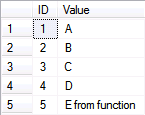UPDATE:

```UPDATE [chv]
SET [chv].[Value] = 'C updated'
FROM [dbo].[ft_ChangeValue]() [chv]
WHERE [ID] = 3
GO

SELECT * FROM [dbo].[SampleTable]
GO```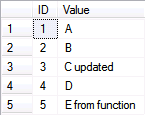DELETE:

```DELETE FROM [dbo].[ft_ChangeValue]()
WHERE [ID] = 2
GO

SELECT * FROM [dbo].[SampleTable]
GO```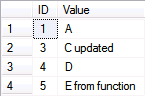One question can be obvious: How this works with parameters? Pretty well.

We will modify our function to include @ID as a parameter and filter dbo.SampleRows by this value:

```CREATE FUNCTION [dbo].[ft_ChangeValue](
@ID INT
)
RETURNS TABLE
AS
RETURN
(
SELECT [ID], [Value]
FROM [dbo].[SampleTable]
WHERE ID = @ID
)
GO```

And DML operations:

INSERT:

```SET IDENTITY_INSERT [dbo].[SampleTable] ON

INSERT INTO [dbo].[ft_ChangeValue](NULL) (ID, VALUE)
VALUES (5, 'E from function with parameter')

SET IDENTITY_INSERT [dbo].[SampleTable] OFF
GO

SELECT * FROM [dbo].[SampleTable]
GO```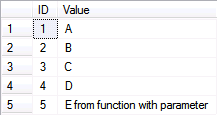Instead of the NULL parameter value we can use any value but it has no effect on INSERT operation. With UPDATE and DELETE operation there is more fun and @ID value is really useful:

UPDATE:

```UPDATE [chv]
SET [chv].[Value] = 'C updated from function with parameter'
FROM [dbo].[ft_ChangeValue](3) [chv]
GO

SELECT * FROM [dbo].[SampleTable]
GO```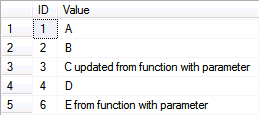DELETE:

```DELETE FROM [dbo].[ft_ChangeValue](2)
GO

SELECT * FROM [dbo].[SampleTable]
GO```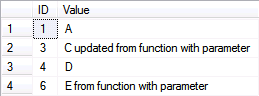Conclusion: Yes, it works. BUT: For 99% of people it will be surprise to see this to be used. It is not intuitive enough and I will say slightly against the nature of the SQL standards. Plus we are limited there by lack of implementation of few features we can use with views like WITH CHECK OPTION.